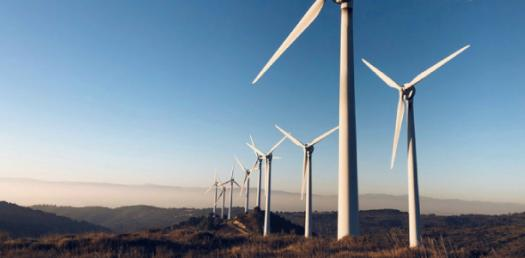# Mechanical Energy Quiz Questions And Answers

10 Questions | Attempts: 1616SettingsA popular quiz on mechanical energy forms is here for you. Answer these mechanical energy quiz questions and answers, and you will not only evaluate yourself, but you will also get a chance to expand your knowledge of different energy forms and what their purpose is. So, take this challenge and score at least 70 percent on this quiz. Are you ready for this challenge? Let's go then, and all the best!

• 1.
A ball is at the top of a ramp. It has not started rolling. What type(s) of energy does it have?
• A.

Kinetic Energy

• B.

Potential Energy

• C.

Gravitational Potential Energy

• D.

Heat Energy

• E.

GPE and KE

• 2.
A ball is rolling down a ramp. It is halfway between the top and the bottom of the ramp. What kind(s) of energy does it have?
• A.

Kinetic Energy

• B.

Potential Energy

• C.

Gravitational Potential Energy

• D.

Heat Energy

• E.

GPE and KE

• 3.
A ball is rolling down a ramp. At what part of the ramp does it have the most kinetic energy?
• A.

The top of the ramp

• B.

The middle of the ramp

• C.

The bottom of the ramp

• D.

After it leaves the ramp and rolls across the floor

• E.

It does not have kinetic energy.

• 4.
A ball is rolling down a ramp. At what part of the ramp does it have the most speed?
• A.

The top of the ramp

• B.

The middle of the ramp

• C.

The bottom of the ramp

• D.

After it leaves the ramp and rolls across the floor

• E.

It does not have kinetic energy.

• 5.
A pendulum is swinging through the air. With each swing, it slows down. What is happening?
• A.

The energy is being lost.

• B.

The energy is transferring into heat energy.

• C.

Kinetic energy is transferring into the air.

• D.

KE is transforming into heat energy and transferring into the air.

• E.

The pendulum is gaining potential energy.

• 6.
What things affect the amount of kinetic energy an object has?
• A.

Distance and time

• B.

Weight and distance

• C.

Mass, height, and speed

• D.

Mass and height

• E.

Mass and speed

• 7.
What things determine how much gravitational potential energy an object has?
• A.

Distance and time

• B.

Weight and distance

• C.

Mass, height, and speed

• D.

Mass and height

• E.

Mass and speed

• 8.
Which of the following is the best example of an object with GPE?
• A.

A car that is driving down the street.

• B.

A battery

• C.

The sun

• D.

A person standing on a ladder

• E.

An object that just stopped moving

• 9.
Which of the following is the best example of an object with potential energy (not gravitational)?
• A.

A car that is driving down the street.

• B.

A battery

• C.

The sun

• D.

A person standing on a ladder

• E.

An object that just stopped moving

• 10.
Which of the following is the best example of an object with kinetic energy?
• A.

A car that is driving down the street.

• B.

A battery

• C.

The sun

• D.

A person standing on a ladder

• E.

An object that just stopped moving

## Related TopicsBack to top
×

Wait!
Here's an interesting quiz for you.